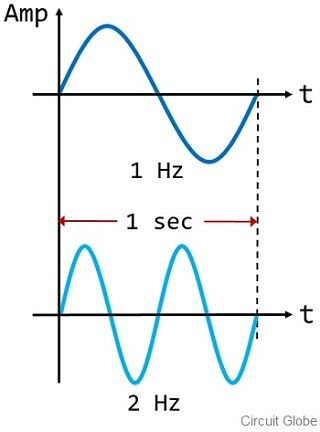# Difference Between Frequency and Bandwidth

Both frequency and bandwidth are the two major terms related to data transmission. The major difference between frequency and bandwidth is that frequency shows the number of complete cycles appearing in unit time. As against bandwidth is the overall amount of data transmitted in a unit time.

Both frequency and bandwidth have a similar measuring unit i.e., hertz. But the two have different meanings when studied in the context of networking. In this article, we will see how bandwidth is different from frequency.

## Comparison Chart

Basis for ComparisonFrequencyBandwidth
BasicIt defines the number of complete cycles in unit time.It defines the amount of data transmitted in unit time.
Specified in terms ofcycles/secbits/sec
Denoted asfB

### Definition of Frequency

Frequency is defined as the total number of wave cycles present in each second of a waveform. It basically shows the occurrence of overall complete wave cycles in the unit time. The figure below represents two different waveforms:Here as we can see that both the waveforms are of different frequencies.

It is clear from the figure that the wavelength of the waveform is larger in the first case while it is quite smaller in the second case. This represents that the two signal waveforms have different frequencies.

As in the first case, the total complete cycle is only 1 per second. While in the second the overall complete cycle is 2 per second. Thus the frequency of the first signal is 1 Hz. Whereas that of the second signal is 2Hz. Hence we can say that the frequency of a signal is dependent on the occurrence of the cycle in unit time.

### Definition of Bandwidth

Bandwidth is completely related to frequency but definitely, bandwidth is not frequency. However, bandwidth is the range of frequencies. Bandwidth is defined as the difference in the upper and lower frequency components present in a signal. Thus, it specifies the amount of data being transmitted per second.

The figure below shows the bandwidth of a signal ‘f’ with lower and upper frequencies as fl and fu respectively:Basically, we know that a signal which is transmitted from an end to another consists of various frequency components. Then among the various frequencies how can we specify the range occupied by the complete signal?

As bandwidth provides an idea about the range of the transmitted signal. So, the range of the signal is determined by calculating the difference between the lowest and highest frequency component present in the signal.

Suppose a signal which is to be transmitted has different frequencies such as 6000MHz, 4200MHz, 1500 MHz, and 820 MHZ. So, here the signal bandwidth will be specified by the difference of the lowest and highest frequency component.

Bandwidth = 6000 MHz – 820 MHz = 5180 MHz

This shows that the signal with a frequency ranging between 6000 and 820 MHz will get transmitted. Thus the bandwidth will be 5180 MHz. It is to be noted that the audible frequency of a human ear is 20 Hz to 20KHz. The sound above 20KHz is known as ultrasonic sound. While the sound below 20Hz is known as infrasonic sound.

## Key Differences Between Frequency and Bandwidth

1. The frequency of a signal defines the total number of complete cycles of a waveform that are existing per sec. While bandwidth is the range of frequency of signal while transmission thus shows its capacity of data flow.
2. The frequency of a signal is specified as cycles/second. While bandwidth is generally specified in terms of bits/sec.
3. To denote frequency lower case letter ‘f’ is used. Whereas bandwidth is denoted by the upper case letter ‘B’.

### Conclusion

So, from this discussion, we can conclude that both frequency and bandwidth are two inter-related but different terms used in networking.